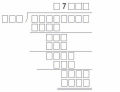證明平行

證明平行

，三角形ACI
1和BCI2 的外心分別為O1和O2 。試證：I1I2 平行O1O2

[ 本帖最後由 tsyr 於 2014-6-17 08:18 PM 編輯 ]

TOP

 lyingheart 萊因哈特發短消息 加為好友 當前離線 2# 大 中 小 發表於 2014-6-18 05:33  只看該作者 先證明A、B、 $$I_1$$ 、 $$I_2$$ 共圓 令三角形ABC的內心為 $$I$$ 因為三角形AFE和ABC相似，且相似比為 $$\cos{A}$$ 令 $$r_1$$ 、 $$r$$ 分別為三角形AFE和ABC的內切圓半徑 那麼 $$\displaystyle r_1=r\cos{A}$$ $$\displaystyle IA=\frac{r}{\sin{\frac{A}{2}}}$$ $$\displaystyle I_1A=\frac{r_1}{\sin{\frac{A}{2}}}=\frac{r\cos{A}}{\sin{\frac{A}{2}}}$$ $$\displaystyle II_1 \times IA=\frac{r^2(1-\cos{A})}{\sin^2{\frac{A}{2}}}=2r^2$$ 同理  $$\displaystyle II_2 \times IB=2r^2$$ 故結論成立 所以若 $$I_3$$ 為三角形CDE的內心，那麼 $$C、I_3、I_1、A$$ 以及 $$C、I_3、I_2、B$$ 共圓 所以 $$O_1O_2$$ 和 $$CI_3$$ 垂直 又 $$\angle{II_1I_2}=\angle{IBA}=\frac{1}{2}\angle{ABC}$$ 且 $$\angle{I_1II_3}=90^o+\frac{1}{2}\angle{ABC}$$ 所以$$I_1I_2$$ 和 $$CI_3$$ 垂直 故得證 [ 本帖最後由 lyingheart 於 2014-6-18 05:21 PM 編輯 ] UID1430 帖子93 閱讀權限10 在線時間262 小時 註冊時間2013-4-24 最後登錄2020-7-17  查看詳細資料 TOP
 tsyr發短消息 加為好友 當前離線 3# 大 中 小 發表於 2014-6-18 18:36  只看該作者 太神了! 如何能夠想到 先證明共圓 呢(因為它要證明平行，很難想到和共圓有關)方便說明並引導一下，解題的思路歷程or訣竅嗎? 因為自己做題目時，都無法自己找到正確的方向。 還是多謝老師了 UID1737 帖子216 閱讀權限10 在線時間176 小時 註冊時間2014-6-7 最後登錄2018-6-29  查看詳細資料 TOP

回復 3# tsyr 的帖子

TOP

 tsyr發短消息 加為好友 當前離線 5# 大 中 小 發表於 2014-6-19 05:31  只看該作者 謝謝老師 UID1737 帖子216 閱讀權限10 在線時間176 小時 註冊時間2014-6-7 最後登錄2018-6-29  查看詳細資料 TOP
﻿